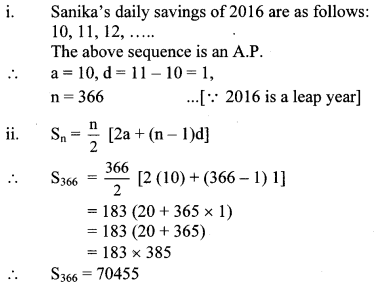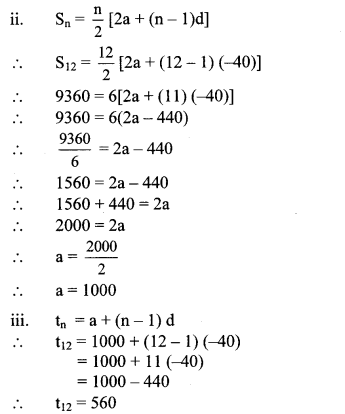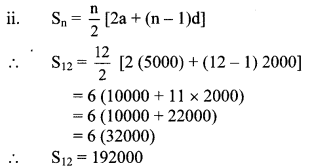# Maharashtra Board 10th Class Maths Part 1 Practice Set 3.4 Solutions Chapter 3 Arithmetic Progression

Balbharti Maharashtra State Board Class 10 Maths Solutions covers the Practice Set 3.4 Algebra 10th Class Maths Part 1 Answers Solutions Chapter 3 Arithmetic Progression.

## Practice Set 3.4 Algebra 10th Std Maths Part 1 Answers Chapter 3 Arithmetic Progression

Question 1.
On 1st Jan 2016, Sanika decides to save ₹ 10, ₹ 11 on second day, ₹ 12 on third day. If she decides to save like this, then on 31st Dec 2016 what would be her total saving?
Solution:∴ Sanika’s total saving on 31st December 2016 would be ₹ 70455.

Question 2.
A man borrows ₹ 8000 and agrees to repay with a total interest of ₹ 1360 in 12 monthly instalments. Each instalment being less than the preceding one by ₹ 40. Find the amount of the first and last instalment.
Solution:
i. The instalments are in A.P.
Amount repaid in 12 instalments (S12)
= Amount borrowed + total interest
= 8000 + 1360
∴ S12 = 9360
Number of instalments (n) = 12
Each instalment is less than the preceding one by ₹ 40.
∴ d = -40∴ Amount of the first instalment is ₹ 1000 and that of the last instalment is ₹ 560.

Question 3.
Sachin invested in a national saving certificate scheme. In the first year he invested ₹ 5000, in the second year ₹ 7000, in the third year ₹ 9000 and so on. Find the total amount that he invested in 12 years.
Solution:
i. Amount invested by Sachin in each year are as follows:
5000, 7000, 9000, …
The above sequence is an A.P.
∴ a = 5000, d = 7000 – 5000 = 2000, n = 12∴ The total amount invested by Sachin in 12 years is ₹ 1,92,000.

Question 4.
There is an auditorium with 27 rows of seats. There are 20 seats in the first row, 22 seats in the second row, 24 seats in the third row and so on. Find the number of seats in the 15th row and also find how many total seats are there in the auditorium?
Solution:
i. The number of seats arranged row-wise are as follows:
20, 22, 24,
The above sequence is an A.P.
∴ a = 20, d = 22 – 20 = 2, n = 27

ii. tn = a + (n – 1)d
∴ t15 = 20 + (15 – 1)2
= 20 + 14 × 2
= 20 + 28
∴ t15 = 48
∴ The number of seats in the 15th row is 48.∴ Total seats in the auditorium are 1242.

Question 5.
Kargil’s temperature was recorded in a week from Monday to Saturday. All readings were in A.P. The sum of temperatures of Monday and Saturday was 5°C more than sum of temperatures of Tuesday and Saturday. If temperature of Wednesday was -30° Celsius then find the temperature on the other five days.
Solution:
Let the temperatures from Monday to Saturday in A.P. be
a, a + d, a + 2d, a + 3d, a + 4d, a + 5d.
According to the first condition,
(a) + (a + 5d) = (a + d) + (a + 5d) + 5°
∴ d = -5°
According to the second condition,
a + 2d = -30°
∴ a + 2(-5°) = -30°
∴ a – 10° = -30°
∴ a = -30° + 10° = -20°
∴ a + d = -20° – 5° = – 25°
a + 3d = -20° + 3(- 5°) = -20° – 15° = -35°
a + 4d = -20° + 4(-5°) = -20° – 20° = -40°
a + 5d = -20° + 5(-5°) = -20° – 25° = -45°
∴ The temperatures on the other five days are
-20°C, -25° C, -35° C, -40° C and -45° C.

Question 6.
On the world environment day tree plantation programme was arranged on a land which is triangular in shape. Trees are planted such that in the first row there is one tree, in the second row there are two trees, in the third row three trees and so on. Find the total number of trees in the 25 rows.
Solution:
i. The number of frees planted row-wise are as follows:
1,2,3,…
The above sequence is an A.P.
∴ a = 1, d = 2 – 1 = 1,n = 25∴ The total number of trees in 25 rows are 325.

Scroll to Top